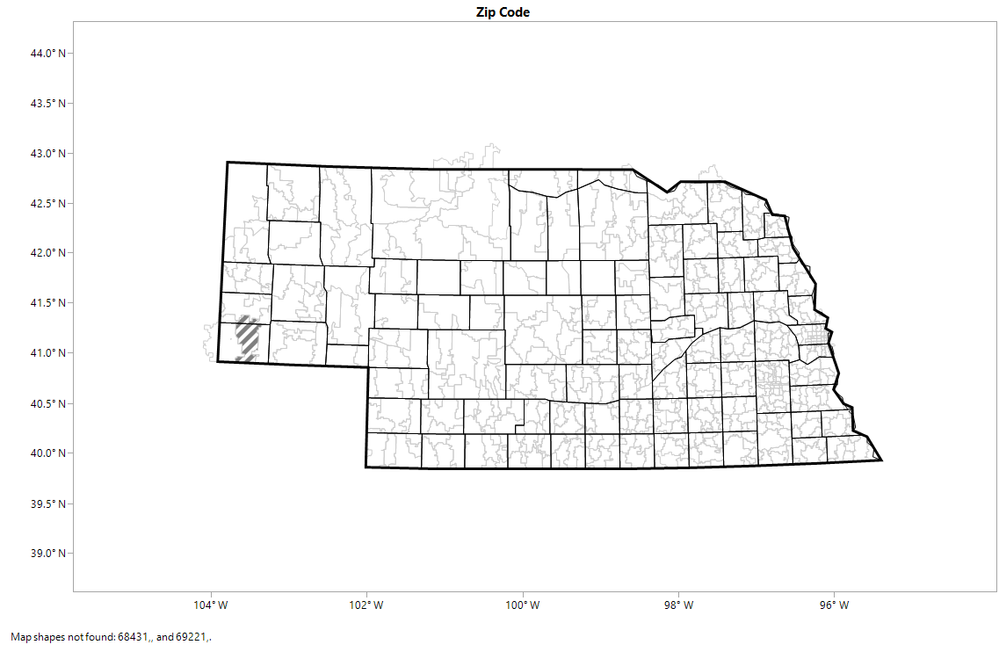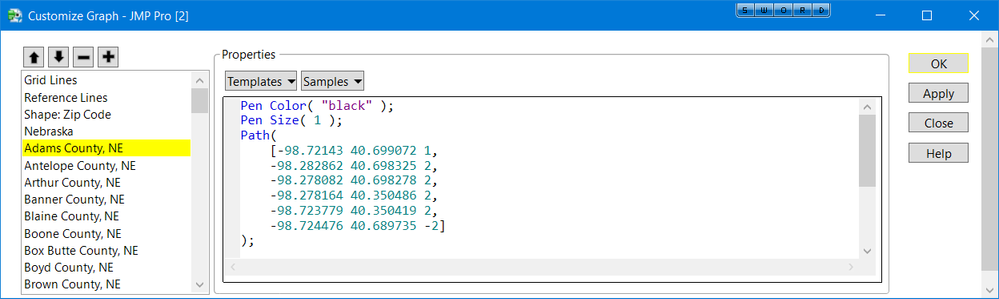Choose Language Hide Translation Bar
Highlighted

## How to add more than one boundary to background map?

Is there any way to add more than one boundary (e.g., state, county, zip code) to the background map which can be individually customized (color, thickness, etc.)?

2 REPLIES 2
Highlighted

## Re: How to add more than one boundary to background map?

I believe, Graph Builder only allows one Map variable. However Supose you have a table of addresses and associated with each address is a zipcode, a county, the state and other pertinet information houshold type, political affiliation, employment status, etc.

Using Craig Hale's code to convert geo spatial info into shape files here is what I would do:

• drag zipcode to the Map area
• add graphic scripts to draw the state outine,
• add a graphic script for each county outline
• make a local graphics filter for zipcode, and county and state

I don't have such a file. I have a data table for the state of Nebrasks zipcode and population that I will use for demo purposes. As stated in other posts, zipcodes can cross county lines (ie not nested), so I cannot in all cases assign a zipcode to a county. But here are a couple of screen shots to show the feasibility. The light gray lines delineate zip codes, the black lines are counties and the thick line is the state outline.

I attached the file with embedded script.``````Names Default to Here(1);

dt = current data table();

gb = dt << Graph Builder(
Variables( Shape( :Zip Code ) ),
Elements( Map Shapes( Legend( 2 ) ) )
);

mloc = Get Path Variable("JMP_Home");
temp = Open( mloc || "Maps/US-State-Name.jmp", Invisible);
idx = temp << get rows where(:Name == "Nebraska");
sid = :Shape ID[idx];
close(temp, NoSave);

temp = Open( mloc || "Maps/US-State-XY.jmp", Invisible);
idx = temp << get rows where(:Shape ID == sid);
sxmat = temp:X[idx];
symat = temp:Y[idx];
Close(temp, NoSave);

spath = concat(sxmat,symat);
nr = nrow(spath);
spath = concat(spath, J(nr,1,2));
spath[1,3]=1;
spath[nr,3] = -2;

Pen Color( "black" );
Pen Size( 3 );
Path(Expr(spath)))
));

//get each county's outline and add to the graph
temp = Open( mloc || "Maps/US-County-Name.jmp", Invisible);
idx = temp << get rows where( ends with(:Full Name Short, "NE") );
sid = :Shape ID[idx];
cname = :Full Name Short[idx];
close(temp, NoSave);

temp = Open( mloc || "Maps/US-County-XY.jmp", Invisible);
for(i=1, i<=nrow(sid), i++,
idx = temp << get rows where(:Shape ID == sid[i]);
cxmat = temp:X[idx];
cymat = temp:Y[idx];

cpath = concat(cxmat,cymat);
nr = nrow(cpath);
cpath = concat(cpath, J(nr,1,2));
cpath[1,3]=1;
cpath[nr,3] = -2;

Pen Color( "black" );
Pen Size( 1 );
Path(Expr(cpath)))
));
); //end for i

Close(temp, NoSave);

``````
Highlighted

## Re: How to add more than one boundary to background map?

Thanks @gzmorgan0

Article Labels